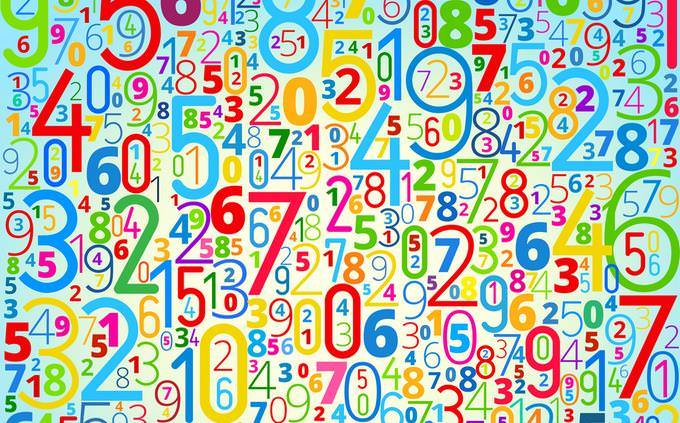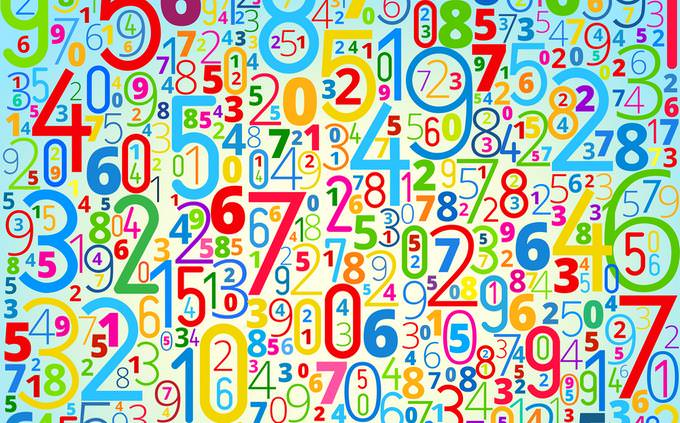# Can You Solve These 15 Math Questions?

Are you ready for another math challenge? This test has a bit of everything, and will require you to recall some of your school-day math skills. Good luck!Can you solve each question in 60 seconds?
Of Course! Let's GoIf a = -4 and b = -2 - What is |a+b|?
6
-6
2
-4Kate took a total of 9 pages of notes during 3 hours of class. In all, how many hours will Kate have to spend in class before she will have a total of 12 pages of notes in her notebook?
4
5
6
7What is 1.248 X (10 to the power of 4)?
1248
12,480
124.8
122,248Ysabel is baking blueberry pies to sell at her bakery. She starts with 8 5/6 cartons of blueberries, of which she uses 5/8 for the first batch of pies. How many cartons of blueberries does Ysabel use for the first batch of pies?
5 and 25/48
2 and 14/22
4 and 21/48
57 + 1 x 1 + 8 ÷ 2 = ?
7
8
12
9Which of these numbers is NOT divisible by 3 (without a remainder)?
162
171
213
212Scott and Lisa each made a bowl of punch. Lisa used 8 1/6 times as much lemonade as Scott did. If Scott used 1/2 of a cup of lemonade, how many cups of lemonade did Lisa use?
4 and 1/12
5 and 1/12
6 and 1/6
4 and 1/67 + 1 x 1 + 8 ÷ 2 = ?
12
7
8
105 + 1 x 0 + 8 ÷ 2 = ?
9
6
7
8Simplify the following formula: 4a + 6b - 4b
4a - 2b
2a - b
4a + 2b
2a + 2bIf X=4, what is the value of 8(2x-4)?
32
24
16
06x - 10 = 4(x+3) - solve for x.
x = 9
x = 8
x = 12
x = 11Find 3 consecutive numbers, with 3 times the first being equal to 8 more than the sum of the other 2 numbers. (x = y+z+8)
11,12,13
2,3,4
8,9,10
13,14,15The ratio of two mountains is 7 to 5. The first mountain is 2100 feet tall. How tall is the second mountain?
1450 Feet
1600 Feet
1650 Feet
1500 FeetWhich of these number is divisible by 4?
7850
780
554
80,742That's a Fail
Unfortunately, you got 1/5 or less of the answers right. That means you still have a lot of practice to do if you want to regain your old math skills. It seems that you've already lost some of them and that's a shame. Why not take a gander at the answers below and take more of our math quizzes to learn and practice your math some more?Below Average
Unfortunately, while not the lowest grade, you did not manage to answer at least half of the questions correctly. While you may have some basic math in place, there is still much to be done for your "mathematical thinking". The best thing for that? Practice, practice, practice! Have a look below at your answers and the correct ones, then try some of our other math quizzes to practice some more.Above Average
Good work! You definitely have your mathematical bases covered, and that is rare for most adults these days that have at least a few decades between them and school. You have a good head for mathematical thinking and so you easily recalled a lot of the methods needed to solve these questions. Nicely done!You ACED IT!
Your mathematical prowess is without fault. You have an uncanny memory for the math skills you learned at school. The more years that have passed between you and high school, the more impressed we are! You obviously have a brain that is good at processing and remembering mathematical information. It's a rare skill and one you should cherish and continue to develop. Practice some more with out other math quizzes. If you've had any mistakes, have a look below to see them corrected.
1 2 3 4 5 6 7 8 9 10 11 12 13 14 15 16
Rate: